Next: Decision Fusion Up: Detection Algorithm Previous: Temporal Wavelet Analysis

## Spatial Wavelet Analysis

The fourth step of our fire detection algorithm is the spatial wavelet analysis of moving regions containing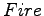mask pixels to capture color variations in pixel values. In an ordinary fire-colored object there will be little spatial variations in the moving region as shown in Fig.(a). On the other hand, there will be significant spatial variations in a fire region as shown in Fig.(a). The spatial wavelet analysis of a rectangular frame containing the pixels of fire-colored moving regions is performed. The images in Figs.(b) and(b) are obtained after a single stage two-dimensional wavelet transform that is implemented in a separable manner using the same filters explained in Subsection. Absolute values of low-high, high-low and high-high wavelet subimages are added to obtain these images. A decision parameter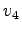is defined for this step, according to the energy of the wavelet subimages: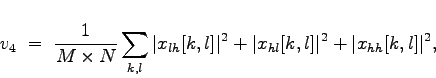(4)

where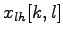is the low-high subimage,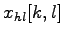is the high-low subimage, and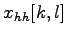is the high-high subimage of the wavelet transform, respectively, and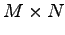is the number of pixels in the fire-colored moving region. If the decision parameter of the fourth step of the algorithm,, exceeds a threshold, then it is likely that this moving and fire-colored region under investigation is a fire region.

Both the 1-D temporal wavelet analysis described in Subsectionand the 2-D spatial wavelet analysis are computationally efficient schemes because a multiplierless filter bank is used for both 1-D and 2-D wavelet transform computation [9,14]. Lowpass and highpass filters have weights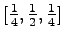and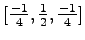, respectively. They can be implemented by register shifts without performing any multiplications.

The wavelet analysis based steps of the algorithm are very important in fire and flame detection because they distinguish ordinary motion in the video from motion due to turbulent flames and fire.Next: Decision Fusion Up: Detection Algorithm Previous: Temporal Wavelet Analysis
ugur toreyin 2005-11-27